Grouped Frequency Distribution Table - What are Class Intervals - Statistics, Class 9, Mathematics

# Grouped Frequency Distribution Table - What are Class Intervals - Statistics, Class 9, Mathematics - Extra Documents & Tests for Class 9

GROUPED DATA
To put the data in a more condensed form, we make groups of suitable size, and mention the frequency of each group. Such a table is called a grouped frequency distribution table.

Class-Interval : Each group into which the raw data is condensed, is called a class-interval.
Each class is bounded by two figures, which are called class limits. The figure on the left side of a class is called its lower limit and that on its right is called its upper limit.

Types of Grouped Frequency Distribution
1. Exclusive Form (or Continuous Interval Form) : A frequency distribution in which the upper limit of each class is excluded and lower limit is included, is called an exclusive form.

Ex. Suppose the marks obtained by some students in an examination are given.
We may consider the classes 0 – 10, 10 – 20 etc. In class 0 – 10, we include 0 and exclude 10. In class 10
- 20, we include 10 and exclude 20.

2. Inclusive Form (or Discontinuous Interval Form) : A frequency distribution in which each upper limit as well as lower limit is included, is called an inclusive form. Thus, we have classes of the form 0 – 10, 11 – 20, 21 – 30 etc. In 0 – 10, both 0 and 10 are included.

Ex.1 Given below are the marks obtained by 40 students in an examination :

3, 25, 48, 23, 17, 13, 11, 9, 46, 41, 37, 45, 10, 19, 39, 36, 34, 5, 17, 21, 39, 33, 28, 25, 12, 3, 8, 17, 48, 34, 15, 19, 32, 32, 19, 21, 28, 32, 20, 23.

Arrange the data in ascending order and present it as a grouped data in :
(i) Discontinuous Interval form, taking class-intervals 1 – 10, 11 – 20, etc.
(ii) Continuous Interval form, taking class-intervals 1 – 10, 10 – 20, etc.

Sol. Arranging the marks in ascending order, we get:
3, 3, 5, 8, 9, 10, 11, 12, 13, 15, 17, 17, 17, 19, 19, 19, 20, 21, 21, 23, 23, 25, 25, 28, 28, 32, 32, 32, 33, 34, 34, 36, 37, 39, 39, 41, 45, 46, 48, 48.
We may now classify them into groups as shown below:

(i) Discontinuous Interval Form (or Inclusive Form)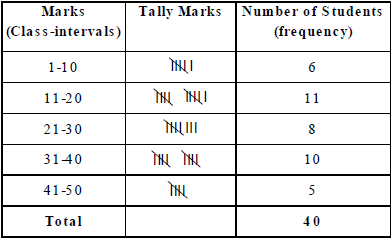Note that the class 1 – 10 means, marks obtained from 1 to 10, including both.

(ii) Continuous Interval Form (or Exclusive Form)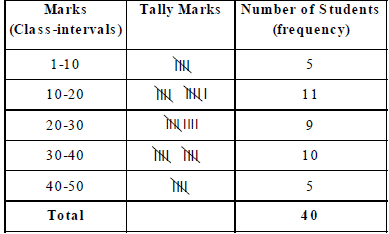Here, the class 1 – 10 means, marks obtained from 1 to 9, i.e., excluding 10.

IMPORTANT TERMS RELATED TO GROUPED DATA

1. Class Boundaries Or True Upper And True Lower Limits :

(i) In the exclusive form, the upper and lower limits of a class are respectively known as the true upper limit and true lower limit.

(ii) In the inclusive form, the number midway between the upper limit of a class and lower limit of the subsequent class gives the true upper limit of the class and the true lower limit of the subsequent class ..
Thus, in the above table of inclusive form, we have :
true upper limit of class 1 – 10 is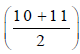= 10.5, and, true lower limit of class 11 – 20 is 10.5.

Similarly, true upper limit of class 11 – 20 is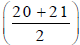= 20.5, and, true lower limit of class 21 – 30 is 20.5.

2. Class Size : The difference between the true upper limit and the true lower limit of a class is called its class size.

3. Class Mark of A Class =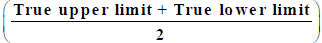The difference between any two successive class marks gives the class size.

Ex.2 The class marks of a frequency distribution are 7, 13, 19, 25, 31, 37, 43. Find the class-size and all the class-intervals.
Sol.
Class size = Difference between two successive class-marks = (13 – 7) = 6.
Let the lower limit of the first class interval be a. Then, its upper limit = (a + 6).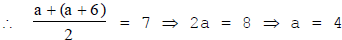So, the first class-interval is 4 – 10.
Let the lower limit of last class-interval be b.
Then, its upper class limit = (b + 6).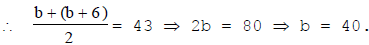So, the last class-interval is 40 – 46.
Hence, the required class-intervals are 4 – 10, 10 – 16, 16 – 22, 22 – 28, 28 – 34, 34 – 40 and 40 – 46.

METHOD OF FORMING CLASSES OF A DATA

1. Determine the maximum and minimum values of the variate occurring in the data.
2. Decide upon the number of classes to be formed.
3. Find the range, i.e., the difference between the maximum value and the minimum value. Divide the range by the number of classes to be formed to get the class-size.
4. Be sure that there must be classes having minimum and maximum values occurring in the data.
5. By counting, we obtain the frequency of each class.

Ex.3 The water tax bills (in rupees) of 30 houses in a locality are given below :

147, 167, 136, 178, 175, 116, 155, 121, 115, 156, 176, 141, 189, 167, 177, 208, 212, 143, 203, 210, 188, 178, 212, 118, 197, 145, 134, 133, 196, 185.

Construct a frequency-distribution table with class-size 10.

Sol. Minimum observation = 115, Maximum observation = 212.
Range = (Maximum observation) – (Minimum observation) = (212 – 115) = 97.
Class size = 10.
Since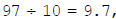, we should have 10 classes, each of size 10. These classes are:
115 – 125, 125 – 135, 135 – 145, 145 – 155, 155 – 165, 165 – 175, 175 – 185, 185 – 195, 195– 205 and 205 – 215.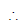The frequency distribution table may be presented as shown below :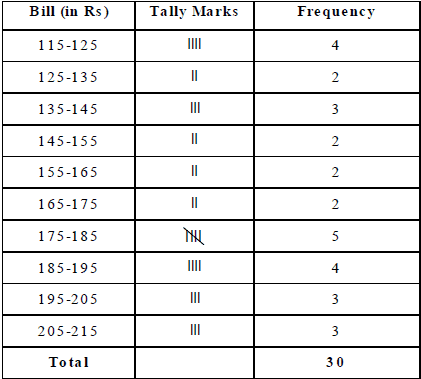RULE TO CONVERT DISCONTINUOUS (OR INCLUSIVE) FORM TO CONTINUOUS (OR EXCLUSIVE) FORM

In a discontinuous interval or inclusive form, we have :

Adjustment factor = (1 over 2)[(Lower limit of one class – Upper limit of previous class)]

Thus, if the classes are 1 – 10, 11 – 20, etc., then adjustment factor = (1 over 2) (11 – 10) = 0.5.

To convert data given in discontinuous form to the continuous form, we subtract the adjustment factor from each lower limit and add the adjustment factor to each upper limit to get the true limits.

Ex. 4 Convert the following frequency distribution from discontinuous to continuous form: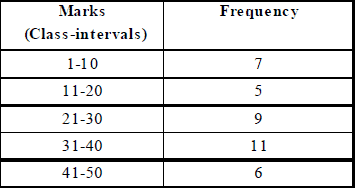Sol. Adjustment factor = (1 over 2)(11 – 10) = 0.5. Subtract 0·5 from each lower limit and add 0·5 to each upper limit.
Then, the required table in continuous form may be prepared as under :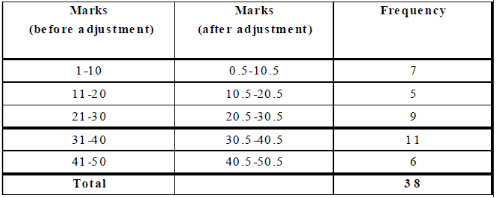CUMULATIVE FREQUENCY OF A CLASS-INTERVAL
The sum of the frequencies of all the previous classes and that particular class, is called the cumulative frequency of the class.

Cumulative Frequency Table
A table which shows the cumulative frequencies over various classes is called a cumulative frequency distribution table.

Ex.5 Following are the ages (in years) of 360 patients, getting medical treatment in a hospital.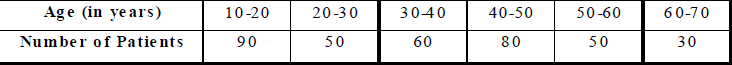Construct the cumulative frequency table for the above data.

Sol. The cumulative frequency table for the above data is given below.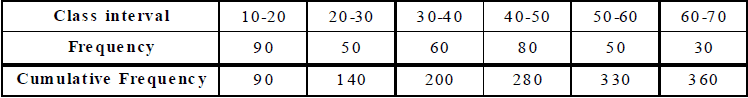This table may be presented in 'less than form', as under.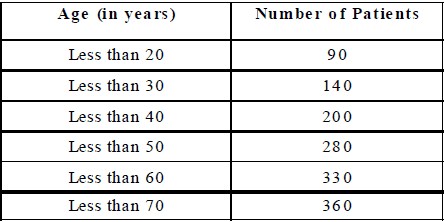Ex.6 The monthly wages (in rupees) of 28 labourers working in a factory, are given below .-

220, 268, 258, 242, 210, 267, 272, 242, 311, 290, 300, 320, 319, 304, 302, 292, 254, 278, 318, 306, 210, 240, 280, 316, 306, 215, 256, 328.

Form a cumulative frequency table with class intervals of length 20.

Sol. We may form the table as under :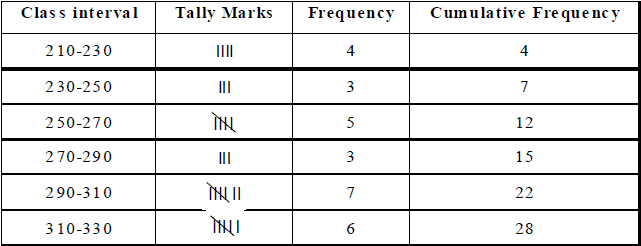The document Grouped Frequency Distribution Table - What are Class Intervals - Statistics, Class 9, Mathematics | Extra Documents & Tests for Class 9 is a part of the Class 9 Course Extra Documents & Tests for Class 9.
All you need of Class 9 at this link: Class 9

## Extra Documents & Tests for Class 9

1 videos|228 docs|21 tests

## FAQs on Grouped Frequency Distribution Table - What are Class Intervals - Statistics, Class 9, Mathematics - Extra Documents & Tests for Class 9

 1. What are class intervals in a grouped frequency distribution table?Ans. Class intervals in a grouped frequency distribution table are the ranges of values that are used to divide a large set of data into smaller, more manageable groups. These intervals can be of equal or unequal size and are used to organize data into a table that shows the frequency of occurrence of each interval.
 2. What is the purpose of a grouped frequency distribution table?Ans. The purpose of a grouped frequency distribution table is to simplify large sets of data into smaller, more manageable groups. This allows for easier analysis and interpretation of the data. The table shows the frequency of occurrence of each interval, which can be used to identify patterns and trends in the data.
 3. How are class intervals determined in a grouped frequency distribution table?Ans. Class intervals can be determined in a number of ways, depending on the data being analyzed. One common method is to use equal intervals, where the range of values is divided into equal-sized intervals. Another method is to use unequal intervals, where the intervals are based on the distribution of the data. In either case, the intervals should be chosen so that each interval contains a sufficient number of data points.
 4. What is the difference between a grouped frequency distribution table and a simple frequency distribution table?Ans. A simple frequency distribution table shows the frequency of occurrence of each individual value in a data set. A grouped frequency distribution table, on the other hand, shows the frequency of occurrence of each interval of values. The grouped table is used when the data set is large and contains many individual values, making it difficult to analyze and interpret.
 5. How can a grouped frequency distribution table be used in statistical analysis?Ans. A grouped frequency distribution table can be used in statistical analysis to identify patterns and trends in the data. By analyzing the frequency of occurrence of each interval, researchers can identify the most common ranges of values in the data set. This information can be used to make predictions and draw conclusions about the data. Additionally, the table can be used to calculate measures of central tendency and variability, such as the mean and standard deviation.

## Extra Documents & Tests for Class 9

1 videos|228 docs|21 testsExplore Courses for Class 9 examSignup to see your scores go up within 7 days! Learn & Practice with 1000+ FREE Notes, Videos & Tests.
10M+ students study on EduRev
Track your progress, build streaks, highlight & save important lessons and more!
Related Searches

,

,

,

,

,

,

,

,

,

,

,

,

,

,

,

,

,

,

,

,

,

,

,

,

,

,

,

;